# The equity premium puzzle and the risk free rate puzzle

It took me some time to understand the relationship between these two puzzles in the lecture material. Essentially, it can be best seen, assuming CRRA preferences, i.e. when individuals have a utility function of the form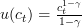$u(c_t) = \frac{c_t^{1-\gamma}}{1-\gamma}$. In this small note, I would like to show the relationship between these two puzzles on basis of CRRA preferences.

With CRRA preferences, the elasticity of intertemporal substitution is merely the inverse of the coefficient of relative risk aversion, denote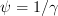$\psi = 1/\gamma$.

This close relationship imposes a problem in some settings, because risk aversion is a concept independent of time. It tells us, how agents deal with uncertainty accross states. Intertemporal substitution is a concept independent of risk considerations. This is a short-comming of CRRA preferences, which is overcome by the usage of Epstein-Zin preferences, which essentially separate these two concepts.  Bansal and Yaron (2002) try to rationalize the equity premium puzzle and the risk-free rate with this more general utility function.

But this is just a side-note.

What does the Equity Premium Puzzle state?

Essentially, what we want to explain are the observed equity premia in the capital markets. The equity premia correspond to the difference between the expected returns on risky assets as compared to a risk free asset.

The Consumption CAPM essentially gives the following formula: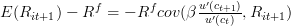$E(R_{it+1}) - R^{f} = - R^{f}cov(\beta\frac{u'(c_{t+1})}{u'(c_t)},R_{it+1})$

Intuitively, the equity premium is givenby the covariance between consumption growth and returns. If consumption growth is positively correlated with returns, then this will imply a negative covariance beween the ratio of marginal utility and thus imply a positive equity premium. In this case, the positive correlation between consumption growth and the returns imply, that the particular asset$i$ works opposite of an insurance as it pays well in good times and is likely to pay bad, in bad times. So, in order to induce agents to hold this asset, the premium has to be positive.

Even more intuitive: we can consider the covariance of consumption with asset returns to be the quantity of risk, whereas the price of risk is given by the coefficient of relative risk aversion.

Empirically, we observe a low correlation between consumption and asset returns. In order to explain the high equity premia, this would imply that we need a high price of risk, i.e. a high RRA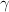$\gamma$.

Now the CRRA preferences come into play, as the Elasticity of Intertemporal substitution is the inverse of the RRA. So a high RRA implies a low EIS.

But what does a low EIS imply?

If agents have a low elasticity of intertemporal substitution, they prefer a flat consumption path. To see this, we need to know a bit more about CRRA preferences. Namely, we need to know how the consumption path changes in case of interest rate changes; any change of interest rates induces a substitution and an income effect. We have three cases:

• If the EIS is equal to 1, so this corresponds to the case of logarithmic utility, then the substitution and the income effect cancel each other out.
• If the EIS is more than 1, i.e. if$\gamma<1$, then the substitution effect is stronger than the income effect, so this implies we substitute current consumption for future consumption in case the interest rates increases.
• If EIS is less than 1, so if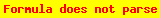$\gamma > 1$, then the income effect dominates, implying that a rise in r will yield to a higher current consumption.

So in this setting a large$\gamma > 1$ implies that the EIS is less than 1, so the income effect dominates; it implies that if we expect (exogenously given) growth in consumption, that we will increase curent consumption to smooth consumption over our lifetime. The exogenously given growth in consumption can be rationalized via simple growth models with exogenous technological progress.

However, if our income currently is fixed, then we can only increase current consumption by borrowing. The risk free rate puzzle now says that, if we have a high RRA, then this implies we should see an increase in borrowing at time t, due to the consumption smoothing induced by the income effect in the presence of expected growth in consumption. However, this would lead to an increase in the risk free interest rate at time t and thus, to a drop in the observed equity premia. However, the low risk free interest rates as e.g. measured by the real return on T-Bills, reject this setting.

So considering the above relationship from the C-CAPM, what we would observe is that with a high$\gamma$, we would have under CRRA preferences the effect that the LHS difference is vanishing, but now, we have a mismatch stemming from the RHS, as the$R^f$ is blown up.